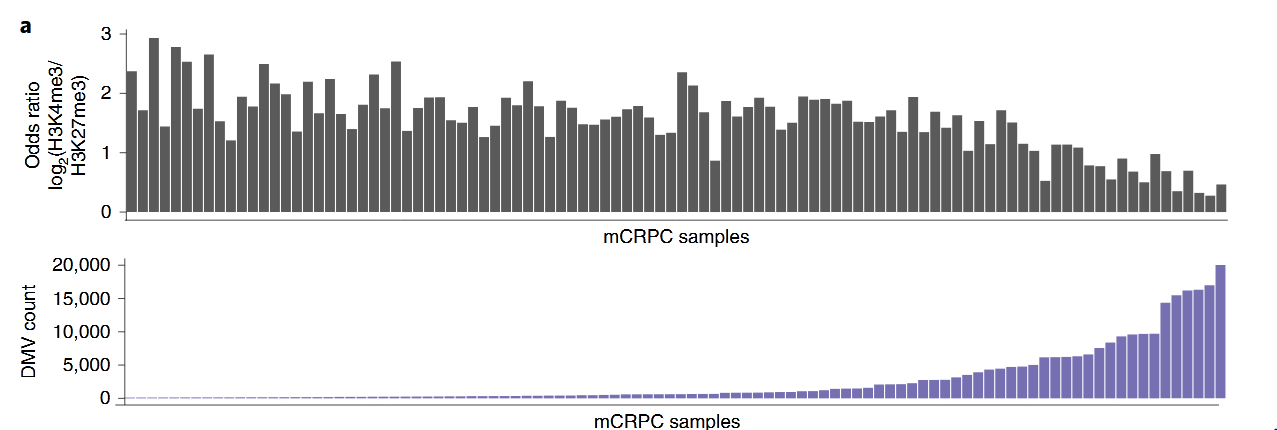# Bar graph of the relationship between the amount of DNA methylation valleys and H3K4me3 and H3K27me3

#### Introduction

DNA methylation valleys (DNA methylation valleys, DMV) are a wide range of hypomethylated regions, which are related to the activation of histone marker H3K4me3 and the inhibition of histone marker H3K27me3. In metastatic castration-resistant prostate cancer (mCRPC, metastatic castration-resistant prostate cancer), as shown in the figure, the greater the number of DMVs in the sample, the higher the signal ratio of H3K4me3 to H3K27me3.Sample-level log2(OR) calculated from the number of DMVs that overlap H3K4me3 vs. H3K27me3 sites. Lower values favor H3K27me3 and higher values favor H3K4me3. Lower: Sample-level count of DMVs, order matching upper panel.

#### Code explanation

``````#Loop through HMRs per sample and assign them to groups
output <- data.frame()
samples2use <- sample_ids_wgbs
colnames(valleydf) <- c('chr','start','end','sample')
valley <- makeGRangesFromDataFrame(valleydf,keep.extra.columns=T)``````
##### Calculate the number of H3K4me3 and H3K27me3 peaks in the methylation valley, and do fisher test to calculate the OR of their relationship with the methylation valley
``````for(i in 1:length(samples2use)) {
sample <- samples2use[i]
valleyi <- valley[valley\$sample==sample]

valleywidth <- sum(width(valleyi))
output[sample,'Sample'] <- sample  #Sample name
output[sample,'width'] <- valleywidth  # Total width of methylated valley
output[sample,'count'] <- length(valleyi)  # Number of methylated valleys

print(paste(i, output[sample,'count']))

in_h3k4me3 <- countOverlaps(tracks[['pca100_H3K4me3']],valleyi,ignore.strand=T)>0
in_h3k27me3 <- countOverlaps(tracks[['pca100_H3K27me3']],valleyi,ignore.strand=T)>0
in_cpg_island <- countOverlaps(tracks[['cpg_island']],valleyi,ignore.strand=T)>0

count_h3k4me3_valley <- sum(in_h3k4me3)
count_h3k27me3_valley <- sum(in_h3k27me3)
count_h3k4me3_novalley <- sum(!in_h3k4me3)
count_h3k27me3_novalley <- sum(!in_h3k27me3)

tab <- rbind(c(count_h3k4me3_valley,count_h3k4me3_novalley),
ft <- fisher.test(tab)  # Fisher test, whether the signal values of h3k4me3 and h3k27me3 are related to the methylation valley

output[sample,'OR'] <- log2(ft\$estimate)
output[sample,'p'] <- ft\$p.value
}

output <- output[rownames(output) %in% sample_ids_wgbs,]
output\$logfdr <- -log10(output\$fdr)

lvl <- output[order(output\$count),'Sample']
#lvl <- output[order(output\$width),'Sample']
output\$Sample <- factor(output\$Sample,levels=lvl)``````
##### Drawing according to the output matrix, ggarrange layout drawing
``````#Set up data frames for plotting
df1 <- cbind.data.frame(sample=output\$Sample,count=output\$OR,SS=output\$fdr<=0.05)
df3 <- cbind.data.frame(sample=output\$Sample,count=output\$width/HG38_SIZE*100)
df4 <- cbind.data.frame(sample=output\$Sample,count=output\$count)

cols <- brewer.pal(n=4, name='Dark2')
plist <- list()
plist[] <- ggplot(df1,aes(x=sample,y=count))+theme_classic()+geom_bar(stat='identity')+
theme(axis.text.x=element_text(angle=90,hjust=1,vjust=0.5))+ylab('Log2 Odds Ratio')+
scale_fill_manual(values=c('darkgray',cols))
plist[] <- ggplot(df3,aes(x=sample,y=count))+theme_classic()+geom_bar(stat='identity',fill=cols)+
theme(axis.title.x=element_blank(),axis.text.x=element_blank(),axis.ticks.x=element_blank())+
ylab('DMV %Genome')  # This picture is not shown in the article in the end
plist[] <- ggplot(df4,aes(x=sample,y=count))+theme_classic()+geom_bar(stat='identity',fill=cols)+
theme(axis.title.x=element_blank(),axis.text.x=element_blank(),axis.ticks.x=element_blank())+
ylab('DMV Count')

ggarrange(plotlist=plist,ncol=1,nrow=length(plist),heights=c(2,1,1))
ggsave(fn_figure2a,width=15,height=12)
``````

#### Reference

Zhao S G , Chen W S , Li H , et al. The DNA methylation landscape of advanced prostate cancer[J]. Nature Genetics, 2020.

#### Original code

https://github.com/DavidQuigley/WCDT_WGBS/blob/master/scripts/2019_05_15_WGBS_figure_2A.R